# Modulus-argument form of complex numbers

By Martin McBride, 2023-07-23
Tags: argand diagram eulers formula complex power complex root polar coordinates complex modulus complex conjugate
Categories: complex numbers imaginary numbersA complex number consists of two parts, a real part and an imaginary part. We often represent it as an (x, y) point on an Argand diagram.

We can also represent a complex number using polar coordinates, and it turns out this is extremely useful when we look at multiplication and powers of complex numbers.

## Argand diagram

We will start by looking at the complex number z given by: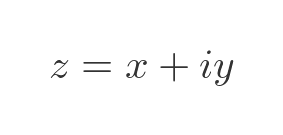Here it is represented on an Argand diagram: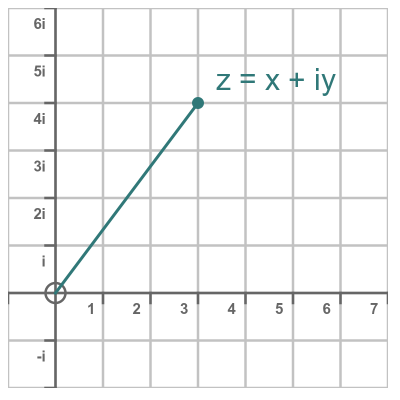In fact, this is the number 3 + 4i, and it is represented by the point (3, 4).

## Polar representation

We can also represent the same number in polar form, also known as the modulus-argument form. This graph shows a complex number in polar form, with the previous (x, y) representation shown alongside it: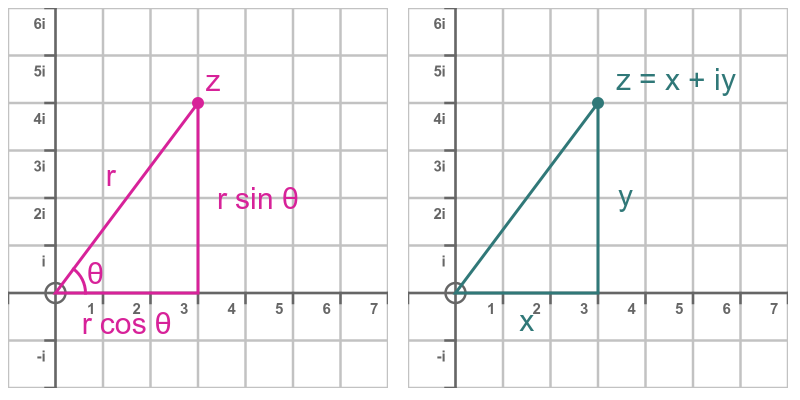The real part of this number is given by: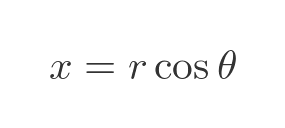The imaginary part is: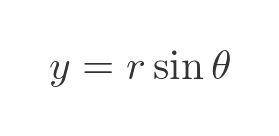So the complex number z can be written in two equivalent ways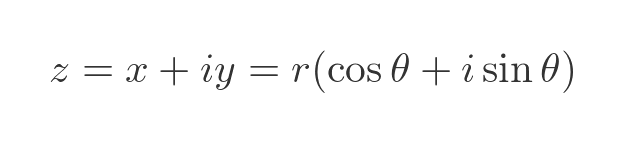The length r is the distance of the point z from the origin. In polar coordinates, this is called the radius of the point, but when talking about complex numbers we usually call it the modulus, denoted |z|.

For complex numbers, the angle Θ is usually called the argument of the number.

We can calculate r from x and y, using Pythagoras' theorem: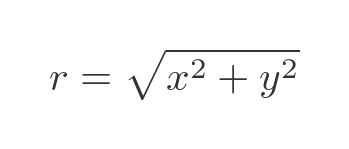We can calculate the angle Θ using the inverse tan function: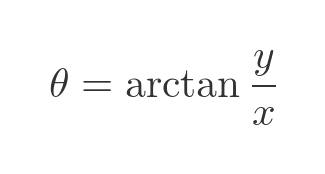## Euler's formula

Euler's formula gives us a way of representing the modulus-argument form of a complex number: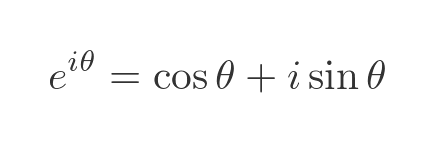Notice that this is e raised to the power of an imaginary number, . It might not be obvious what e raised to an imaginary power is, or what that even means, but Euler's formula answers that question. We won't prove it here, that needs an article of its own. For now, we will assume it is true.

If we multiply both sides by some real number r we get this: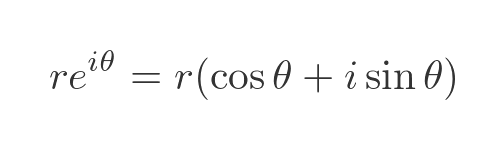Since we already know that the right-hand side of the formula above represents a general complex number z we can therefore write: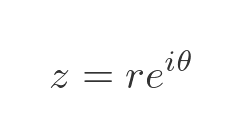## Multiplication of complex numbers using Euler's formula

If we have two complex numbers z1 and z2: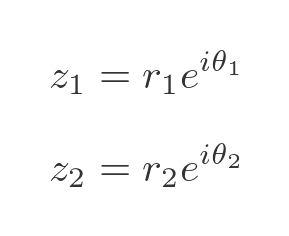The product of these two numbers is: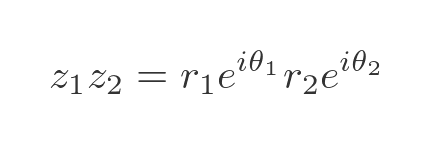We won't prove it here, but it can be shown that imaginary powers of e behave in the same way as real powers. So this simplifies to: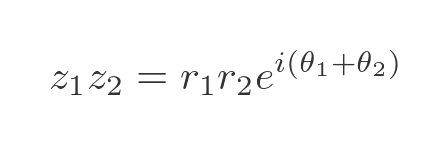So when we multiply two complex numbers, expressed in modulus-argument form, we multiply the moduli (r1 and r2) and add the angles (Θ1 and Θ2). This is indeed what happens, as described in Why does complex number multiplication cause rotation?

Here, the diagram on the left shows z1 and z2 with their angles Θ1 and Θ2. The diagram on the left shows the product of z1 and z2 with the angle Θ1 + Θ2: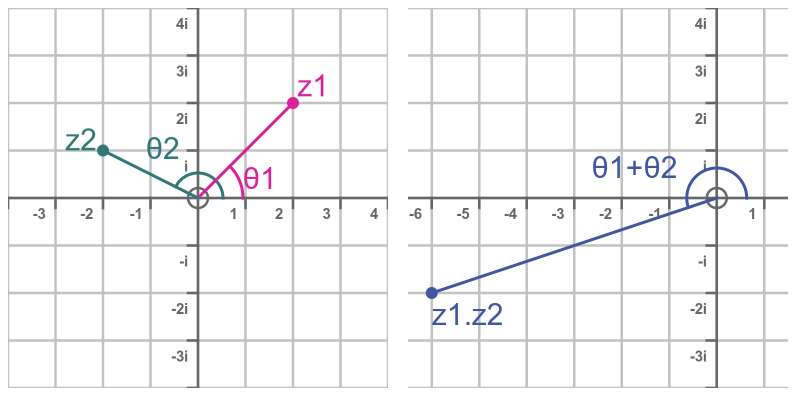## The cyclic nature of the modulus-argument form

In the diagram below, we will multiply two complex numbers as before: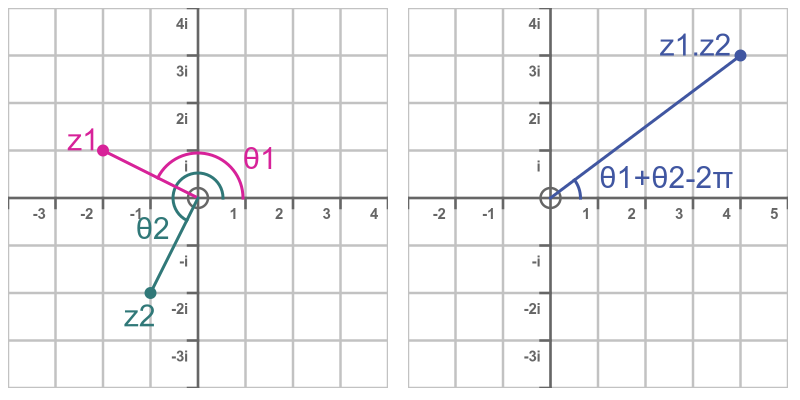In this case, however, the sum of the two angles is greater than 2π. This means that the product z1.z2 wraps all the way around back into the first quadrant. So the final angle Θ1 + Θ2 can alternatively be expressed as Θ1 + Θ2 - 2π. These two angles are equivalent. This is because the modulus-argument form is cyclic.

Why is this? Well, we know that the trig functions sin and cos are cyclic, so we can add or subtract any multiple of 2π from Θ without changing the value of the sin function: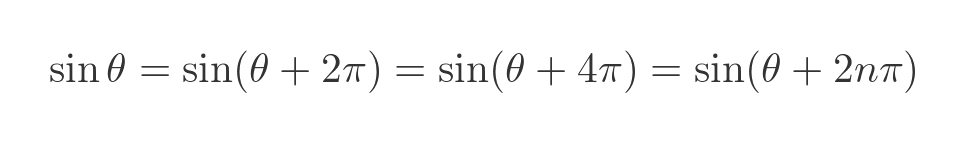The same is true of cos of course. And since Euler's formula is based on sin and cos it follows that: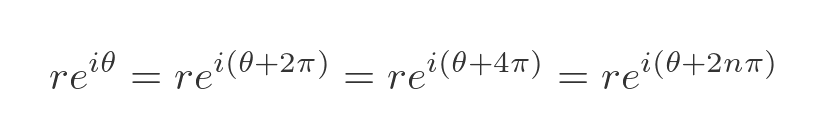We normally express complex numbers using a value of Θ such that π ≤ Θ < π. This value is called the principal argument. Using this rule, our previous multiplication example would look like this: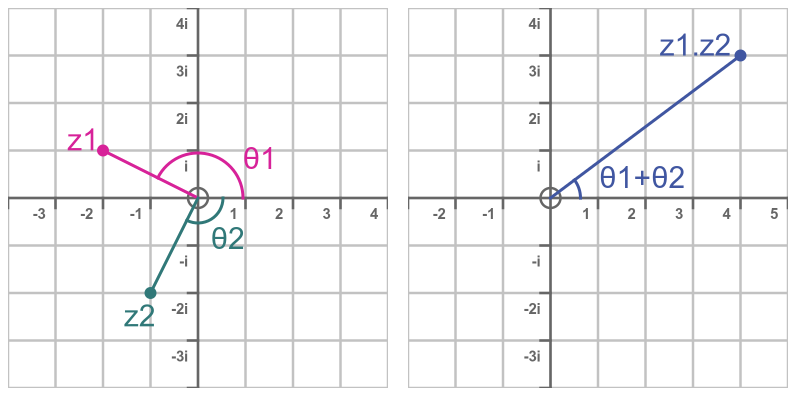Now z2 has a negative angle, so when we add Θ1 and Θ2 we get a small positive result. There is no need to subtract 2π to obtain the correct result. However, when we calculate powers it is sometimes still necessary to adjust the result to find the principal argument, as we will see later.

## Complex conjugate and modulus using Euler's formula

For a complex number z, the complex conjugate z* is formed by negating the imaginary part of z. This is shown here: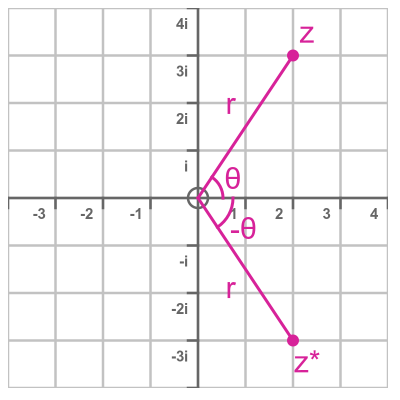The conjugate is rotated by the same angle as z, but in the opposite direction. So the angle of the conjugate is :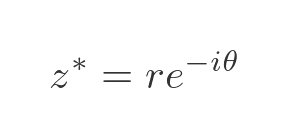This gives us an easy way to express the conjugate in modulus-argument form, we just negate the angle.

If we multiply z and its conjugate, we add the angles, which cancel out to zero: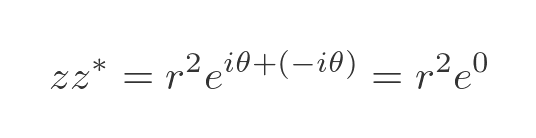Since e to the power 0 is 1 we get a real value, r squared: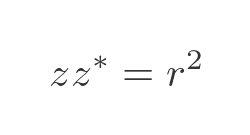And since r is equal to |z| we can find the modulus like this: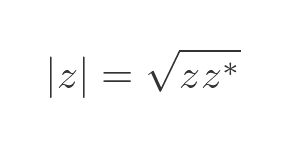## Division of complex numbers using Euler's formula

Taking the same complex numbers z1 and z2 from the multiplication example, here is what complex division looks like: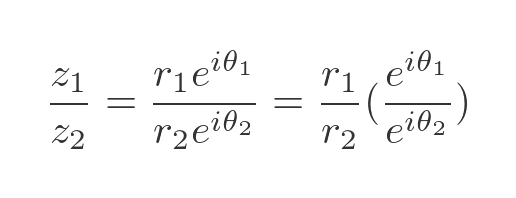We can simplify this by using this rule relating to reciprocals of powers: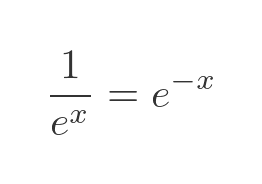Applying this to the exponential term on the bottom gives the following: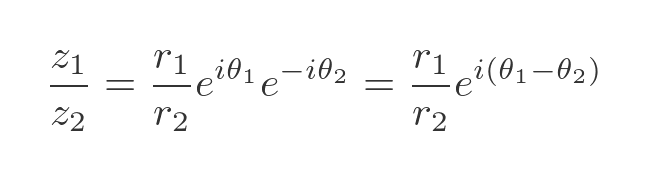So when we divide one exponential by another, expressed in modulus-argument form, we divide the moduli and subtract the angles. Intuitively, this makes sense because division is the opposite of multiplication.

## Powers of complex numbers

We can use the modulus-argument form to calculate a complex number raised to a real power, like this: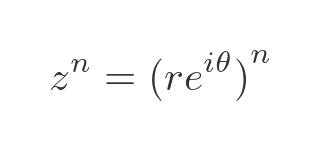We can simplify this using the rule for a power raised to a power: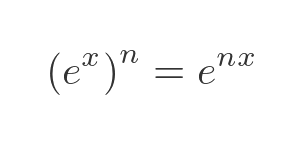This gives us the following formula: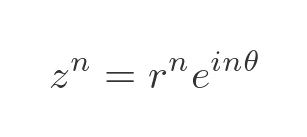So to raise z to the power n we raise the modulus r to the power n and multiply the angle by n. Here is an illustration of cubing the number z: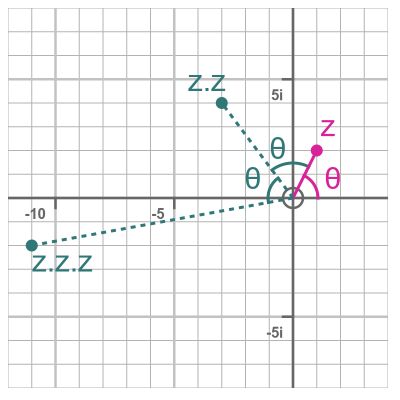The solid line is the value z, with an argument Θ.

The first dotted line represents z squared. The angle is twice the angle of z (an additional angle Θ is added to the angle of z). Its modulus is r squared, where r is the modulus of z.

The second dotted line represents z cubed. It is rotated again by Θ compared to z squared, making its total angle . Its modulus is r cubed.

This works for all real values of n, not just integer values. This means it also works for roots, using the rule that: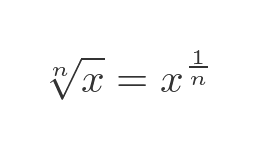This allows us to find the nth of a complex number like this: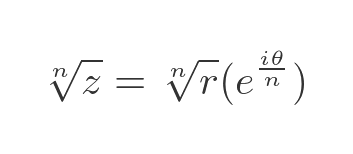In other words, to find the nth root of a complex number expressed in modulus-argument form, we take the nth root of the modulus and divide the angle by n.

## Multiple roots

For real numbers, we know that the square root of a positive number has two values, one positive and one negative. So the square root of 4 can take the value 2 or -2. We also know, of course, that a negative number has no real square root, otherwise we wouldn't be discussing complex numbers in the first place.

The cube root of a real number always has exactly one real value. For example, the cube root of 8 is 2, and the cube root of -8 is -2.

When we consider complex numbers, for positive integer n, the nth root of a number always has exactly n roots (except for the case of z equals zero). For example, the cube root of -8 has 3 values, but 2 of them are complex numbers, so we don't see them when only considering real numbers. The extra roots come from the fact that adding an integer multiple of 2π to the argument of a complex number does not affect its value.

So let's find the cube root of -8. We will express -8 in modulus-argument form: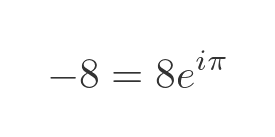This tells us that -8 has a modulus of 8 and an angle of π radians (in other words, -8 it is half a turn counterclockwise from +8). We find the cube root by finding the positive real cube root of 8 (which is 2) and diving the angle by 3, which gives π/3 (a sixth of turn, or 60 degrees, counterclockwise from +2). Here is the equation: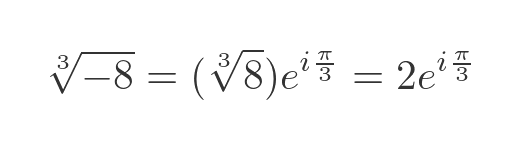But remember that we can add 2π to the argument in the modulus-argument form of -8, which gives an alternative way to express -8 in this form: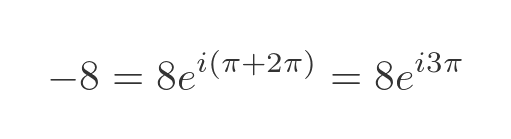This gives an alternative value for the cube root of -8: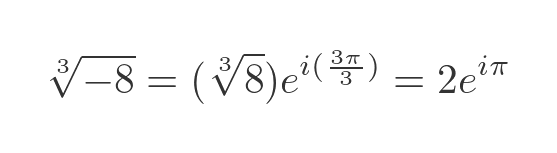This has the same modulus as previously, but an angle of π radians, which means that this root is -2, the only real value root of -8.

We can add another 2π to give yet another definition of -8: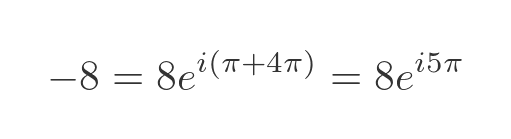This gives yet another value for the cube root of -8: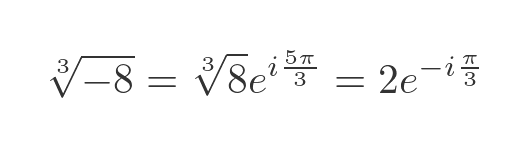The angle here is 5π/3 radians. Since this value is greater than π, we need to normalise it to find the principal argument. Subtracting 2π from the value gives an angle of -π/3.

If we tried this again, adding another 2π to the definition of -8, we would end up with an argument of 7π/3 in the cube root. This normalises to π/3, so it is a duplicate of the first root we found. There are only 3 unique roots.

Here are the 3 cube roots of -8 on an Argand diagram: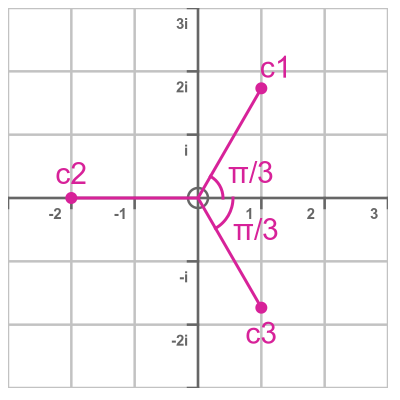The roots all have the same modulus, 2, and they are all separated by the same angle 2π/3. In general, the nth root of a complex number (where n is a positive integer) will have n values that are separated by an angle of 2π/n.